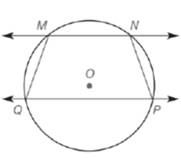Chapter 6.1, Problem 38E### Elementary Geometry for College St...

6th Edition
Daniel C. Alexander + 1 other
ISBN: 9781285195698

#### Solutions

Chapter
Section### Elementary Geometry for College St...

6th Edition
Daniel C. Alexander + 1 other
ISBN: 9781285195698
Textbook Problem
1 views

# If M N ↔ ∥ P Q ↔  in ⊙ O , explain why M N P Q is an isosceles trapezoid.(HINT: Draw a diagonal.)To determine

To explain:

The MNPQ is an isosceles trapezoid by using the provided figure.

Explanation

Given:

The MNPQ, in O shown below.

Postulate used:

Theorem:

The measure of an inscribed angles is equal to the one-half the measure of its intercepted arc.

If AB and BC intersect only at point, then mAB+mBC=mABC.

Calculation:

Since, M and P both are inscribed angles and NPQ and QMN are intercepted arcs.

Since, there is a theorem state that the measure of an inscribed angles is equal to the one-half the measure of its intercepted arc.

M=12(mNPQ)P=12(mQMN)

### Still sussing out bartleby?

Check out a sample textbook solution.

See a sample solution

#### The Solution to Your Study Problems

Bartleby provides explanations to thousands of textbook problems written by our experts, many with advanced degrees!

Get Started

#### Find more solutions based on key concepts